# Approximation by a polynomial with the condition of passing through the points

When simulating data using the least squares method, the curve usually does not pass through the measurement points (Figure 1).

What if you want this curve to pass exactly through one or more highlighted points (Fig. 2 – shown with green circles)?

## 1. Motivation

Let’s consider the usual problem of approximation by a polynomial and see what we are missing in it.

Given N points on the plane (xi, yi) are the values ​​of the unknown function f (x). Find a polynomial p (x) of degree M (M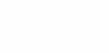It is often assumed that the “best” is the polynomial, the sum of the squares of the deviations which from the given points is minimal: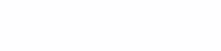In this form, the problem is solved by the well-known least squares method (OLS).

For M i, yi) (fig. 1). Moreover, it often turns out that he does not pass through any of them.

This in itself is not bad. Usually, the data used to construct the approximation contain measurement errors. It is even better that the polynomial does not go through all the points, since this smoothes the measurement errors.

However, it happens that there is more information about the simulated dependency. For example, the response of the sensor to a certain value is simulated. And from physical considerations it is clear that this response is strictly zero when the value is zero. When simulating the characteristics of such a sensor, it may turn out that the approximating polynomial does not pass through zero. As a result, the model will give a large error in the region of zero.

In such a situation, I would like to find a polynomial with the following properties:

1. Pass zero exactly

2. Has the smallest sum of squares of deviations from the rest of the measured values

The article describes a method for finding such a polynomial. Moreover, it is possible to require the exact passage of the polynomial not through one, but several selected points.

## 2. Solution

### 2.1. Weighted Least Squares

As an ingredient we need weighted least squares, which minimizes the sum of squared deviations with weights at each point: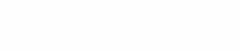Where wi – weighting factors. Usually, weighted least squares are used to take into account measurement errors at each point, therefore, in the literature, not the concept of weight is often used, but the inverse variance σ2 errors: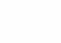Weighted OLS is well described in the book , ch. 15.1, 15.4.

### 2.2. Accurate zero crossing

Let’s start with a simple case: we require the polynomial p (x) to pass through zero exactly.

To do this, we find a polynomial q (x) of degree M-1 by the modified points (xi, yi/ xi) using the weighted least squares method, choosing as weights wi= xi2; and multiply it by x. Then: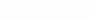It will have degree M and pass zero exactly.

Consider the sum of the squares of its deviations from the remaining points (xi, yi):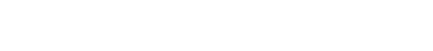But it is this value that is minimized by the weighted LSM over the modified points. Therefore, the polynomial p (x) will also correspond to the second condition of the problem – to have the least sum of squares of deviations from (xi, yi).

### 2.3. Exact passing through one arbitrary point

Let us complicate the problem and require the exact passage of the polynomial p (x) through one arbitrarily given point (ξ, η).

By changing the variables u = x-ξ, v = y-η, the problem is reduced to the one considered above. Find the coefficients of the polynomial p0(u) passing through zero and having the least sum of squares of deviations p0(ui) -vi… Next, let’s move on to the original variables: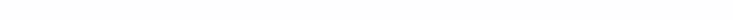To find the coefficients p (x), one can perform algebraic manipulations with the coefficients p0(x-ξ), but there is an easier way in practice. Since we already have an implementation of an ordinary OLS in our program, we can simply calculate the values ​​of p (x) by formula (1) at some M + 1 or more points, and apply the ordinary OLS to these values, setting the degree of the polynomial M. this is how the coefficients p (x) will be found.

### 2.4. Accurate passing through multiple points

And, finally, the most general case – we require the exact passage of the polynomial p (x) through K given points (ξi, ηi). You should choose K ≤ M, otherwise the problem degenerates into interpolation.

Passing to the solution, we introduce a polynomial z (x) of degree K: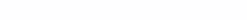It has zeros at the points ξ1, ξ2, … ξK

We also introduce Lagrange interpolation polynomial L (x) of degree K-1 passing through the points (ξi, ηi) , ch. 3.1.

We use the weighted least squares method to find the polynomial q (x) of degree MK from the modified points: (xi, (yi-L (xi)) / z (xi)) with weight coefficients wi= z (xi)2… We obtain the coefficients {aj} for q (x) that minimize the sum: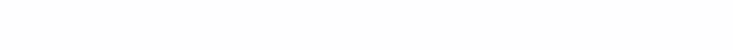Now let’s compose the polynomial p (x) as:Let us prove that p (x) is a solution to the problem.

1. Passage through points (ξi, ηi) is ensured by the fact that z (ξi) = 0, vanishing the first term. The second term at the same points, L (x), passes through (ξi, ηi) by construction.

2. The degree of p (x) is equal to M, since the degree of the product of the polynomials q (x) and z (x) is equal to the sum of the degrees of the factors. In this case, M-K + K = M. The sum of polynomials has the degree of the highest of the terms. The degree of L (x) is equal to K-1, which is less than M.

3. Let us write the sum of the squares of the deviations at the points (xi, yi):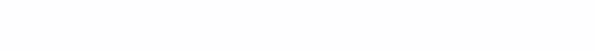But this coincides with the value (2), which was minimized when finding the coefficients q (x). Therefore, p (xi) has the least sum of squares of deviations from yi… Q.E.D.

To find the coefficients p (x), one can perform algebraic manipulations with q (x), z (x), and L (x), but this is even more difficult than in Section 2.3. above. So here it is also recommended to calculate the values ​​of p (x) at M + 1 or more points by formula (3) and apply the usual least squares method to them.

## 3. The price of the issue and recommendations for use

You have to pay for everything in this life. Imposing the condition of exact passage through the points (ξi, ηi) to the polynomial p (x) leads to the fact that the sum of the squares of its deviations from the remaining points (xi, yi) turns out to be, generally speaking, higher than without imposing conditions.

In fact, we have solved the problem of conditional optimization of the sum of squares of deviations p (xi) -yi… As in other problems of conditional optimization, the achieved value of the objective function turns out to be worse than with unconditional optimization. Otherwise, unconditional optimization would give the same result.

When should the described method be applied? Then, when there is “reinforced concrete” information about the modeled dependence that it must pass through the points (ξi, ηi) regardless of the experimental data (xi, yi).

In my practice, there was one such case. The nonlinear characteristic of the sensor was simulated. The true form of functional dependence was unknown. All that remained was to use polynomials in an attempt to somehow compensate for the nonlinearity. But polynomials were poor at describing the characteristic around zero. Obtained by the usual OLS, they tried not to pass through zero. Nevertheless, from physical considerations, it was clear that the characteristic of the sensor must pass through zero. That’s when the conditional imposition came in handy. Its application made it possible to improve the compensation of nonlinearity in the vicinity of zero and to reduce the relative error of the device as a whole.

## 4. An example with specific numbers

This example was used to build the graphs shown in Fig. 1 and fig. 2.

The following polynomial was taken as the “true dependence”: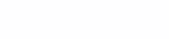Where T3(x) – Chebyshev polynomial third order. Modifications are applied to it in order to display an interesting plot section in the range x, y ∈ [0; 1]… p1(x) goes through points (0,0) and (1,1). Its expansion in powers of x looks like this: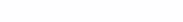The values ​​of p1(x) at 11 equidistant points between 0 and 1 in 0.1 increments. The normally distributed “measurement error” δ was added to the values ​​of the polynomial.i with variance σ2= 0.04. At points x1= 0 and xeleven= 1 no error was added. By random numbers, the cards were laid down as follows:

 i 1 2 3 4 5 6 7 eight nine ten eleven xi 0 0.1 0.2 0.3 0,4 0.5 0.6 0.7 0.8 0.9 1 δi 0 -0.1617 0.0162 0.0294 -0.0458 0.3358 0.2476 -0.1325 -0.3986 -0.4477 0

An approximation by ordinary least squares at given points with M = 3 gave the following coefficients: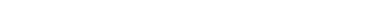Graph p2(x) is shown in Fig. 1. Achieved sum of squares of deviations p2(x) -yi amounted to 0.4019.

For the test described in Sec. 2.4. method, the condition was set for the exact passage of the polynomial through the points (0,0) and (1,1), and at the remaining points – the minimization of the sum of the squares of the deviations. As a result, the following polynomial was obtained: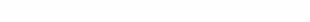Its graph is shown in Fig. 2. Achieved sum of squares of deviations p3(x) -yi amounted to 0.5046.

From the given example, we can conclude that the theoretical calculations were confirmed. The polynomial p was found3(x) approximating “experimental data” and passing through points (0,0) and (1,1). As predicted in Sec. 3, the sum of the squares of its deviations from the points (xi, yi) turned out to be larger than the polynomial p2(x) found by ordinary least squares. However, this sacrifice can be justified if passing p3(x) through points (0,0) and (1,1) is more important.

As for the coincidence of the coefficients of the polynomials p2(x) and p3(x) with the coefficients of the “true” polynomial p1(x) – then on a couple of test data sets, a special pattern was not revealed. It probably makes sense to run a Monte Carlo test and compare the distributions of the coefficients p2(x) and p3(x) on a large sample.

## Conclusion

In the article, a generalization of the least squares method was obtained, which makes it possible to impose on a polynomial the condition of passing through given points.

The problem was reduced to the use of a weighted least squares method and interpolation by a Lagrange polynomial. Since both operations are reduced to solving a system of linear equations, the method described in the article preserves this (low) computational complexity. The exact solution can be obtained in a finite number of actions and (with due diligence or the use of computer algebra systems) expressed analytically.

The method can be generalized for problems of approximation not only by polynomials, but also by other functions. But this topic is vast and can only be considered in separate publications.

## Literature

 William H. Press et al. “Numerical Recipes in C ++: The Art of Scientific Computing”, Second Edition. Cambridge University Press, ISBN 0-521-75033-4